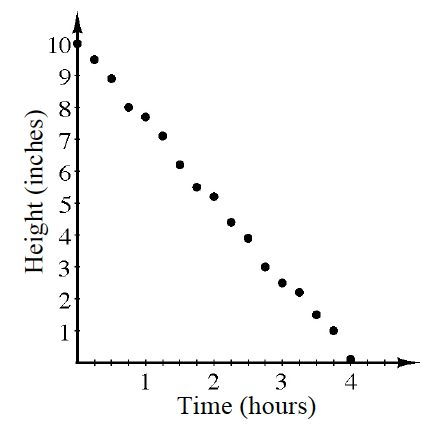### Home > CCA2 > Chapter A > Lesson A.1.2 > ProblemA-27

A-27.

At an aunt’s wedding, Nicolas collected data about an ice sculpture that was about to completely melt. A graph of his data is shown at right.

1. Calculate the equation of a line of best fit.

Use $y=mx+b$.

2. Based on your equation, how tall was the ice sculpture one hour before Nicolas started measuring?

$12.5$ inches